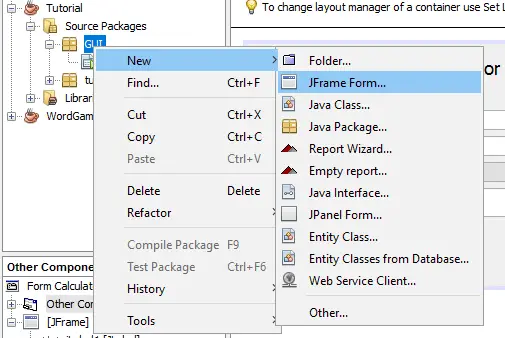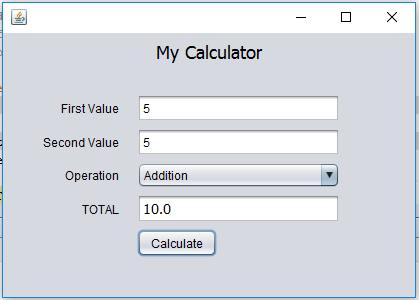# Basic Calculator Using Java with GUI

0
1340

This tutorial will show you on how to Create a Basic Calculator using java with the four mathematical operations. The calculator is designed with GUI (Graphical User Interface).

The calculator uses a JFrame Form with jTextfield, jCombobox, jLablel, and jButton elements located in your Netbeans tool palletes. We also uses “if Statement” to identify the operation before the program calculate the two value. Please follow all the steps to complete this tutorial.

### Basic Calculator in Java with GUI Steps2. Design the JFrameFrom the same with the image below.3. Double click your jButton to automatically generate the button event.

[java]private void jButton1ActionPerformed(java.awt.event.ActionEvent evt) {
} [/java]

4. Insert the code below inside your button event.

double result = (Double.parseDouble(jTextField1.getText()) + Double.parseDouble(jTextField2.getText()));
jTextField3.setText(Double.toString(result));
}else if (jComboBox1.getSelectedItem().equals(“Subtraction”)){
double result = (Double.parseDouble(jTextField1.getText()) – Double.parseDouble(jTextField2.getText()));
jTextField3.setText(Double.toString(result));
}else if (jComboBox1.getSelectedItem().equals(“Multiplication”)){
double result = (Double.parseDouble(jTextField1.getText()) * Double.parseDouble(jTextField2.getText()));
jTextField3.setText(Double.toString(result));
}else if (jComboBox1.getSelectedItem().equals(“Division”)){
double result = (Double.parseDouble(jTextField1.getText()) / Double.parseDouble(jTextField2.getText()));
jTextField3.setText(Double.toString(result));
}[/java]

5. Final Output

## Basic Calculator using Java OutputI hope you might find tutorial helpful.If you have any questions or suggestion about Basic Calculator using Java, Please feel free to contact me on our Contact Page.

Related Tutorial you may like: Basic Calculator Using VB.Net

This site uses Akismet to reduce spam. Learn how your comment data is processed.